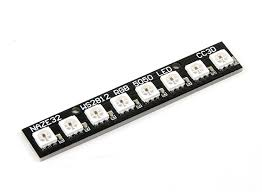# Some Neopixel examples

This example takes the Wemos Mini Ws2812B example a bit further on and use an 8 LED Neopixel strip

Here is an image of the strip we usedLayout

A simple connection required for this one, you connect 3v3 to 5v / Vcc of the strip, Gnd goes to Gnd and you need an I/O line to go to the DIN. I use D8 in the examples below.

Here is a layout to give you the ideaCode Examples

A couple of code examples

 Source code```#include <Adafruit_NeoPixel.h>

#define PIN D8

//the Wemos WS2812B RGB shield has 1 LED connected to pin 2

void setup()
{
pixels.begin(); // This initializes the NeoPixel library.
}

void loop()
{
int led;
for(led=0; led <=8; led++)
{
setColor(led,255,0,0,100); //red
}
for(led=0; led <=8; led++)
{
setColor(led,0,255,255,100); //
}
for(led=0; led <=8; led++)
{
setColor(led,255,0,255,100); //
}
}

//simple function which takes values for the red, green and blue led and also
//a delay
void setColor(int led, int redValue, int greenValue, int blueValue, int delayValue)
{
pixels.setPixelColor(led, pixels.Color(redValue, greenValue, blueValue));
pixels.show();
delay(delayValue);
}```

And another example

 Source code```#include <Adafruit_NeoPixel.h>

#define PIN D8

void setup()
{
pixels.begin(); // This initializes the NeoPixel library.
}

void loop()
{
int led;
for(led=0; led <=7; led++)
{
setColor(led,255,0,0,100); //red
}
for(led=0; led <=7; led++)
{
setColor(led,0,0,0,100); //switch them off
}
}

//simple function which takes values for the red, green and blue led and also
//a delay
void setColor(int led, int redValue, int greenValue, int blueValue, int delayValue)
{
pixels.setPixelColor(led, pixels.Color(redValue, greenValue, blueValue));
pixels.show();
delay(delayValue);
}```

a random color example

 Source code```#include <Adafruit_NeoPixel.h>

#define PIN D8

//the Wemos WS2812B RGB shield has 1 LED connected to pin 2

void setup()
{
pixels.begin(); // This initializes the NeoPixel library.
}

void loop()
{
rndColor(100);
}

//simple function which takes values for the red, green and blue led and also
//a delay
void setColor(int led, int redValue, int greenValue, int blueValue, int delayValue)
{
pixels.setPixelColor(led, pixels.Color(redValue, greenValue, blueValue));
pixels.show();
delay(delayValue);
}

void rndColor(int delayValue)
{
int led = random(0,7);
int redValue = random(0, 255);
int greenValue = random(0, 255);
int blueValue = random(0, 255);
pixels.setPixelColor(led, pixels.Color(redValue, greenValue, blueValue));
pixels.show();
delay(delayValue);
}```
Previous articleWemos Mini Ws2812B example
Next articleWemos OLED shield example